English

# How To: Sort and create a sequentially ordered ID field in an attribute table in ArcGIS Pro

## Summary

Sorting and creating a sequentially ordered ID field in an attribute table in ArcGIS Pro is an efficient way to organize data. The largest and smallest values, or perhaps the most and least significant features in a layer become easily recognizable.

## Procedure

The following steps describe how to sort and create a sequentially ordered ID field in an attribute table in ArcGIS Pro using three different options: the sort ascending or sort descending function, the calculate field function, or a Python script.

Using the Sort Ascending or Sort Descending function

1. Right-click the desired feature to open the Attribute Table.1. Right-click the desired field in the attribute table.
2. Select Sort Ascending, Sort Descending, or Custom Sort. An ordered field is created.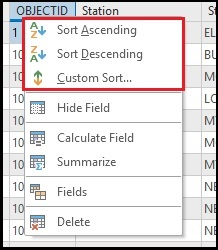Using the Calculate Field function

```Note:
This option only generates sequential numbers for unsorted data based on the OBJECTID order. If the data is sorted, the generated numbers are not sequential.```
1. Open the Attribute Table of the desired feature.
2. Right-click the desired field in the attribute table.
3. Select Calculate Field to open the Calculate Field console.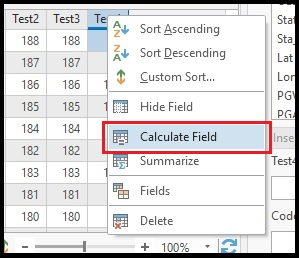1. In the Calculate Field console, click the Helpers filter icon, and select Function.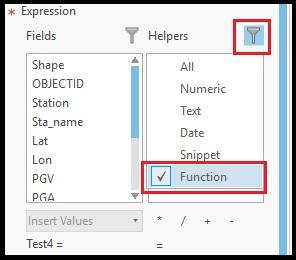1. Double-click the Sequential Number function. This generates the Expression and the Code Block section to create the sequential numbers.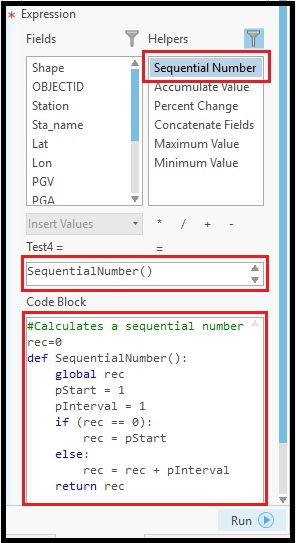1. Click Run. The sequential numbers based on the OBJECTID field is created in the field specified by the Calculate Geometry function.

Using a Python script through the Python console

The following steps demonstrate how to create sorted sequential numbers using the autoIncrement() ArcPy function:

1. Start the Python console. Click the Analysis tab and select Python.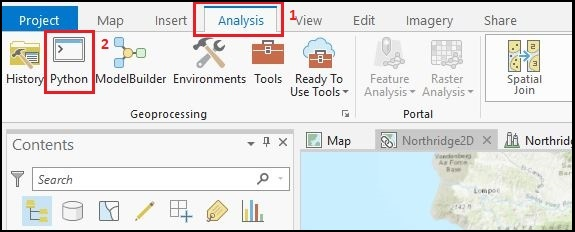1. Import the ArcPy library.
`import arcpy`
1. Set the desired feature, the field to base the order, and the field to populate the sequential numbers.
```sortFeat  = r'[Geodatabase]\[Feature]'
sortField = '[Base Field to sort]'
idField   = '[Field to populate sequential numbers]'```
```Note:
To create the sequential numbers in a new field, create a new field and specify the new field name in the idField segment. For more information on creating a new field, refer to the following web help document, ArcGIS Pro: Add Field.```
1. Define a counter parameter.
`rec = 0`
1. Start the autoIncrement() function.
```def autoIncrement():
global rec
pStart    = 1
pInterval = 1
if (rec == 0):
rec = pStart
else:
rec += pInterval
return rec```
1. Populate the field with the sequential numbers, and delete any extra rows.
```rows = arcpy.UpdateCursor(sortFeat, "", "", "", sortField + " A")

for row in rows:
row.setValue(idField, autoIncrement())
rows.updateRow(row)

del row, rows```

The following code sample demonstrates a full working script.

```import arcpy

sortFeat  = r'C:\Users\\Northridge.gdb\Stations'
sortField = 'OBJECTID'
idField   = 'Station'
rec=0

def autoIncrement():
global rec
pStart    = 1
pInterval = 1
if (rec == 0):
rec = pStart
else:
rec += pInterval
return rec

rows = arcpy.UpdateCursor(sortFeat, "", "", "", sortField + " A")

for row in rows:
row.setValue(idField, autoIncrement())
rows.updateRow(row)

del row, rows```

## Related Information

Last Published: 9/19/2019

Article ID: 000018847

Software: ArcGIS Pro 2.2.4, 2.2.3, 2.2.2, 2.2.1, 2.2, 2.1.3, 2.1.2, 2.1.1, 2.1, 2.0.1, 2.0